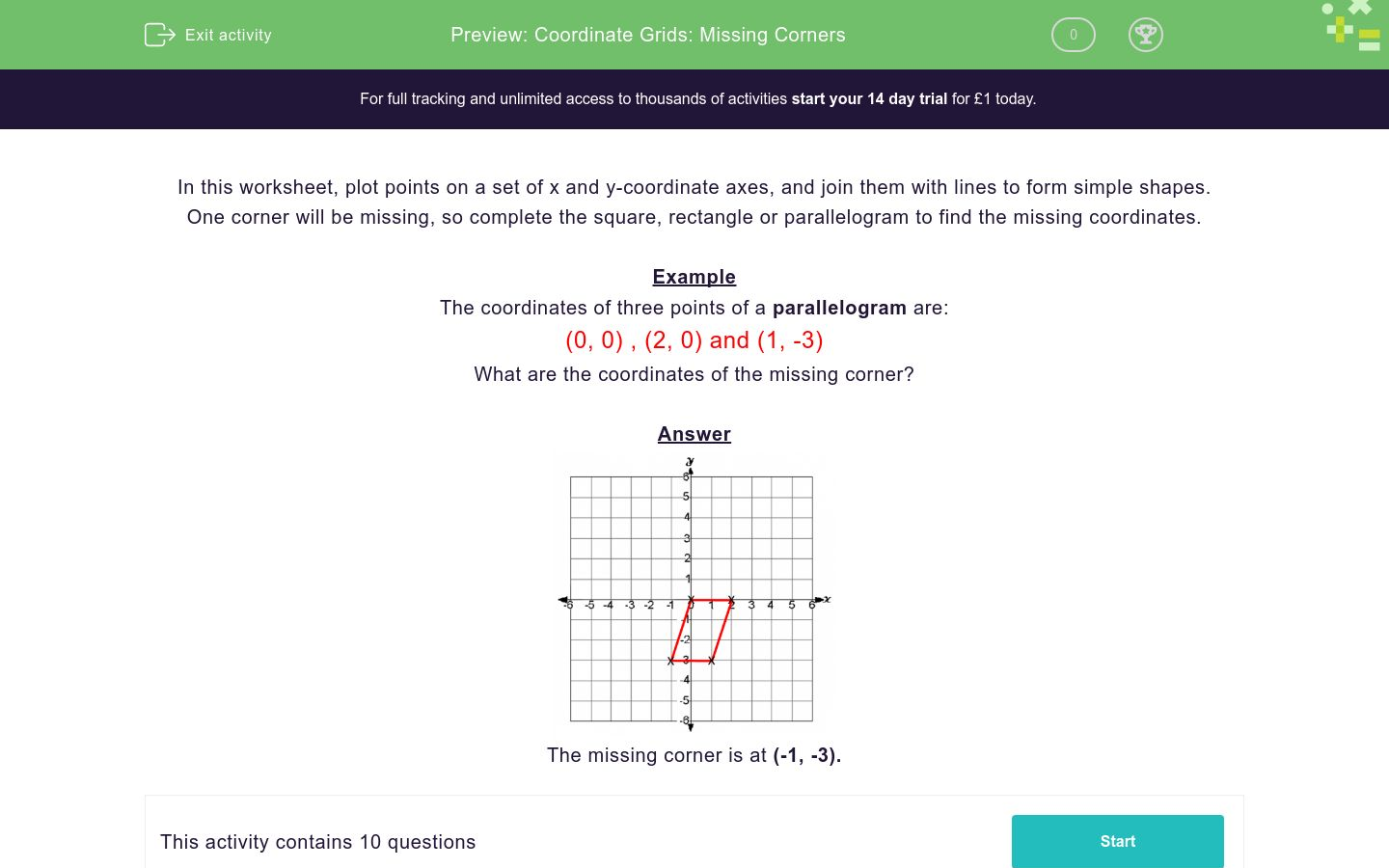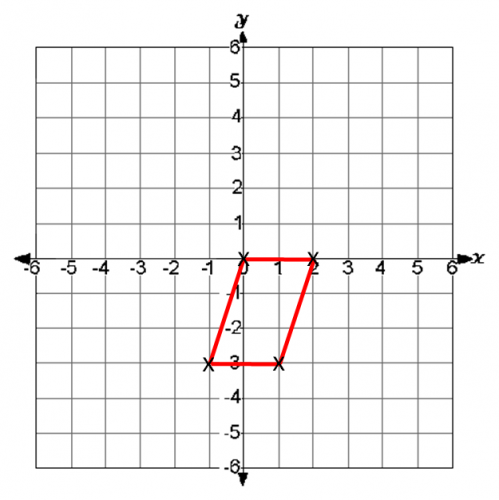# Coordinate Grids: Missing Corners

In this worksheet, students must state the coordinates of the missing corner of the given quadrilaterals.Key stage:  KS 2

Curriculum topic:   Geometry: Position and Direction

Curriculum subtopic:   Draw/Translate Shapes

Difficulty level:#### Worksheet Overview

In this worksheet, plot points on a set of x and y-coordinate axes, and join them with lines to form simple shapes.

One corner will be missing, so complete the square, rectangle or parallelogram to find the missing coordinates.

Example:

The coordinates of three points of a parallelogram are:

(0, 0) , (2, 0) and (1, -3)

What are the coordinates of the missing corner?